# Resistors

Two resistors connected in series give the resulting resistance 65Ω and 10.4Ω in parallel. Determine the resistance of these resistors.

Correct result:

R1 =  52 Ω
R2 =  13 Ω

#### Solution: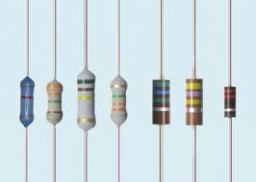We would be pleased if you find an error in the word problem, spelling mistakes, or inaccuracies and send it to us. Thank you!Tips to related online calculators
Looking for help with calculating roots of a quadratic equation?
Do you have a linear equation or system of equations and looking for its solution? Or do you have quadratic equation?

## Next similar math problems:

• Two resistors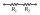Two resistors when they give 25 ohms in series and 4 ohms in parallel what the values of
• Lowest voltage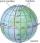Three resistors with resistors R1 = 10 kΩ, R2 = 20 kΩ, R3 = 30 kΩ are connected in series and an external voltage U = 30 V is connected to them. On which resistor is the lowest voltage?
• Two resistorsTwo resistors 20 Ω and 60 Ω are connected in series and an external voltage of 400 V is connected to them. What are the electrical voltages on the respective resistors? Please comment!
• Current in the conductorCalculate the current in the conductor (in mA) if it is connected to a 4.5 V voltage source and its resistance is 20 (ohm).
• ResistanceDetermine the resistance of the bulb with current 200 mA and is in regular lamp (230V).
• The bulbWhat is the resistance of a filament lamp when it is connected to a 9V battery and has a current of 120 mA?
• Mains voltageHow much electric current flows through the appliance with a resistance of 40kΩ, which is connected to the mains voltage (230 V)?
• Electronics: Resistors in parallel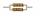From relation for calculating the resistance of parallel combination of resistors: ? Calculate the R, if R1 = 2Ω a R2 = 15Ω
• Effective and mean voltage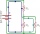A voltage divider consisting of resistors R1 = 103000 Ω and R2 = 197000 Ω is connected to the ideal sine wave voltage source, R2 is connected to a voltmeter which measures the mean voltage and has an internal resistance R3 = 200300 Ω, the measured value i
• Closed circuitIn a closed circuit, there is a voltage source with U1 = 12 V and with an internal resistance R1 = 0.2 Ω. The external resistance is R2 = 19.8 Ω. Determine the electric current and terminal voltage.
• Copper windingCalculate the current flowing through the copper winding at an operating temperature of 70°C. Celsius, if the winding diameter is 1.128 mm and the winding length is 40 m. The winding is connected to 8V.
• The bulbsThe bulbs are connected serially two bulbs and one resistor. Each bulb has a resistivity of 100 ohms and resistor of 60 ohms The voltage source in the circuit is 24 volts. Do you know what voltage to measure on individual appliances?
• Filament of bulbThe filament of bulb has a 1 ohm resistivity and is connected to a voltage 220 V. How much electric charge will pass through the fiber when the electric current passes for 10 seconds?
• Resistance of the resistorThe resistor terminals have a voltage of 20 V and a current of 5 mA is passed through. What is the resistance of the resistor?
• Coil as a girl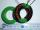The electrical resistance of the copper wire coil is 2.0 ohms. What current runs through the coil when the voltage between the terminals is 3.0 V?
• TransformerSolve the textbook problems - transformer: a) N1 = 40, N2 = 80, U2 = 80 V, U1 =? b) N1 = 400, U1 = 200 V, U2 = 50 V, N2 =?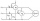Two wattmeters are connected to measuring power in a 3 phase balanced load. Determine the total power and power factor if the two wattmeters read 1000 watts each (1) both positive and (2) second reading is negative